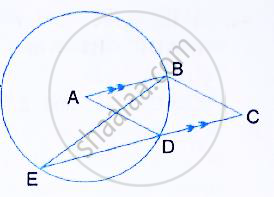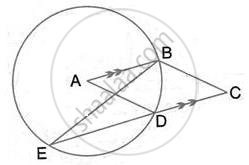Share

# In the Given Figure, a is the Centre of the Circle, Abcd is a Parallelogram and Cde is a Straight Line. Prove that : ∠Bcd = 2∠Abe . - Mathematics

Course

#### Question

In the given figure, A is the centre of the circle, ABCD is a parallelogram and CDE is a straight line.
Prove that : ∠BCD = 2∠ABE .#### Solution(Angle at the centre is double the angle at the circumference subtended by the same chord)
And  ∠BED = ∠ABE (alternate angles)

∴ ∠BAD = 2∠ABE            ……… (i)

ABCD is a parallelogram

∴ ∠BAD = ∠BCD                ………. (ii)

(opposite angles in a parallelogram are equal) From (i) and (ii),
∠BCD = 2∠ABE

Is there an error in this question or solution?